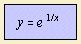# reciprocal exponential function

## exponential

last updated: 2003-08-01

#The curve has asymptotes for x = 1 and y = 0.
It has two parts, the left side in the picture is to be seen in quantum mechanics. Given a set of harmonic oscillators, the proportion of the amount in quantum state n+1 to the amount in state n - as function of the temperature - is given by the curve 1).

The right side can be found (as inverse), for the density of primes 2).

The function is also called the modified exponential function.

Its inverse is the reciprocal logarithm.

notes

1) Kittel 1976 p.128.

2) y = 1 / ln(n), Blij 1990 p.14.